# Types of Linear regression

Hi everybody,

In the previous blog (Introduction to Linear regression), we presented the general definition of Linear regression. In this post, to help you feel more familiar with this charming Machine learning model, we will show you a number of different types of Linear regression based on various points of view.

After reading this post, you will be confident with many variants of Linear regression (LR), you can put your future models to the right category, and reading references about Linear regression will be easier because you have had a firm basis of knowledge.

Do you have enough motivation for reading? If yes, let’s get started!

Types based on the number of predictor variables

As the section’s header suggested, we separate models by the number of predictors each of them uses.

The Linear regression models that use only 1 predictor are called Simple Linear regression, while the models that used at least 2 predictors are called Multiple Linear regression.

Some examples of Simple LR are:

• Using the height of your students to predict their performance in Physical Education.
• Using the number of views on a Youtube video to predict how much the author of that video earns.
• Using the size of a balloon to predict how much it costs.

Well, in the above examples, we use only 1 value to calculate the expected outcome, so they are all called Simple Linear regression.

On the contrary, if we use more than 1 predictor, that will be a Multiple Linear regression:

• Using the temperature and humidity to predict ice-cream sales.
• Using the height and weight of your students to predict their performance in Physical Education.
• Using the number of views and likes and comments on a Youtube video to predict how much the author of that video earns.
• Using the size and material of a balloon to predict how much it costs.

A note on the last example above: the material of a balloon is not a numerical value, it is a categorical value instead (e.g. it can be rubber or plastic, but not 1.0 or 2.0). Hence, we cannot simply apply the normal linear regression formula here (how can we make a combination of a numerical value and a categorical value?). For this case, we have to apply a transformation to convert categorical value to numerical value first, before applying a regression.

To conclude this section, let me write down the formulaic representation of these 2 Linear regression types.

Simple Linear regression: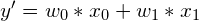.

Multiple Linear regression: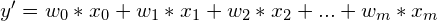where m is the number of predictors,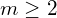.

Recall that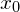is not a predictor, we addto the formula for mathematical convenience, and we always set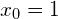.

Types based on the number of response variables

We may ask ourselves: if we can have many predictor variables, can we have many response variables as well?

Yes, we can have more than 1 response variables.

If we have only 1 response variable , our model is called Univariate, while Multivariate is the type of models that have from 2 response variables.

All the examples I have given on this blog up till now are Univariate. So, let me give some examples of Multivariate Linear regression problem:

• Using the temperature and humidity to predict ice-cream sales and the number of customers (1 customer can buy more than 1 cup of ice-cream).
• Using the height of your students to predict their grade in Physical Education of the first semester, the second semester and the overall grade of that school year.

I will skip the formulaic representation of Univariate LR as well. Here is for Multivariate LR: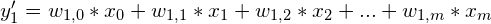.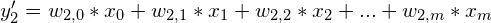.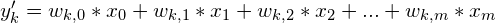.

where m is the number of predictors,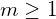,
and k is the number of response variables,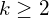.

An observation: the response variables on Multivariate LR can be non-correlated. The weight (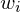) for each response (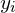) is also separated from the others. So, Multivariate LR is essentially just a group of Univariate LRs.

A final note on this: when browsing the internet, you will likely come across some webpages that mistakenly use the term Multivariate LR to describe Multiple LR. Because cautious on that, don’t be confused!

Types based on Regularization

Regularization is a technique that can be included in our models to prevent them from over-fitting.

Up to now, all the LR formulas I have introduced do not include any regularization.

There are 2 common types of regularization for Linear regression, named L1 (another name is Lasso) and L2 (another name is Ridge).

Hence, your model is called Lasso Regression if it includes Lasso inside, Ridge Regression if it includes Ridge. If you model contains both L1 and L2, it will be called an Elastic Net.

I will leave it there for now, as the explanation of different regularization methods is beyond the scope of this blog. There is another blog dedicated to Regularization.

Conclusion

This is the end. Let me summarize in the following table:

## Types of linear regression

CriteriaValueName
Number of predictors1Simple LR
> 1Multiple LR
Number of responses1Univariate LR
> 1Multivariate LR
Method of RegularizationL1Lasso LR
L2Ridge LR
Both L1 and L2Elastic Net

A final note: notice that the above criteria are NOT mutually exclusive. That means, for example, you can call a model Multivariate Multiple Linear regression if it has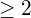predictors andresponse variables.

You can find the full series of blogs on Linear regression here.